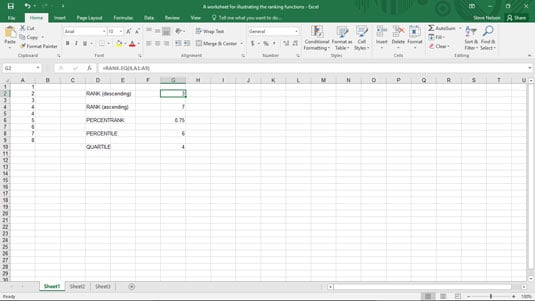##### Excel Data Analysis For DummiesThe PERCENTILE.EXC and PERCENTILE.INC functions in Excel determine the array value at a specified percentile in an array. You use the PERCENTILE.EXC function to determine the percentile exclusive of the first and last values in the array, and you use the PERCENTILE.INC function to determine the percentile inclusive of the first and last values in the array. Both formulas use the same arguments.

The PERCENTILE.EXC formula uses the syntax

`=PERCENTILE.EXC(array,k)`

The PERCENTILE.INC formula uses the syntax

`=PERCENTILE.INC(array,k)`

where array gives the array of values and k gives the percentile of the value that you want to find.

To find the value at the 75-percent percentile in the array of values (inclusive) shown in the worksheet range A1:A9 in this figure, use the formulaA worksheet fragment with the array 1, 2, 3, 4, 4, 5, 6, 7, 8.
`=PERCENTILE.INC(A1:A9,.75)`

The function returns the value 6 because the value 6 is at the 75th percentile in this array. This formula appears in cell G8 in the worksheet shown.

To repeat something in the earlier discussion of the PERCENTRANK function, note that Excel calculates the percentage rank by looking at the number of array values greater than the x value and the number of array values smaller than the x value. For the array shown, the array includes the values 1, 2, 3, 4, 4, 5, 6, 7, 8. The percent rank of 6 in the array equals 0.75 because six array values are smaller than 6 and two array values are larger than 6.